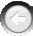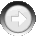﻿ MakeChromosome

# MakeChromosome

Navigation:  »No topics above this level«

# MakeChromosomeMakeChromosome(Population As Integer, Chromosome As Integer, Precision As Integer, MaxValue As Single, MinValue As Single, IntType as Integer)   Purpose: This function creates a continuous (non- integer and integer) chromosome.  Each parameter (independent variable) of the problem corresponds to a chromosome in the individual.  In this function, the user specifies the bit resolution and the maximum and minimum values of the chromosome, as well as the type (continuous/integer) of the chromosome.   Arguments: Population is the number of the population in which you want to create a chromosome. Chromosome is a number of the chromosome that you are going to create.  You may use up to 5000 chromosomes (parameters) in one individual.  Chromosomes are made one by one, and their numbers must be successive.  The first chromosome has the number 0.  New chromosomes can be added at any time. Precision is a number specifying the bit resolution for the chromosome.  Three values are possible: 1 = 8-bit resolution.  The search will be made on the grid consisting of 256 (2^8) points. 2 = 16-bit resolution.  The search will be made on the grid consisting of 65,536 (2^16) points. 3 = 32-bit resolution. The search will be made on the grid consisting of 4,294,967,296 (2^32) points. You may use the symbolic constants BIT8, BIT16, and BIT32 found in GALIB32.BAS to code these precision values. The value of the precision parameter determines the theoretical limit of a solution's accuracy. When rough approximation is enough, a low 8-bit resolution may be used.  When the problem is highly sensitive to small variations in parameters, you may wish to use chromosomes with a higher resolution.  Usually, a 16-bit resolution is appropriate.  Sometimes it is beneficial to compare solutions obtained with different resolutions of the chromosomes. MaxValue is the high limit of a chromosome value.  The search for an optimum value will be conducted up to and including this limit. MinValue is the low limit of a chromosome value.  The search for an optimum value will be performed down to and including this limit. IntType specifies the type of chromosome. IntType=1 will create an integer chromosome; IntType=0 will create a continuous chromosome. In the DLL memory, the integer chromosome is stored as a 16-bit integer number, so the precision parameter plays no part in the integer chromosome.  Instead of precision, you may want to use any dummy integer number. There is no special mapping procedure of the input parameters into integer chromosomes as in the case of continuous ones.  The mins/maxes for the integer chromosome can be any integer values from the range -32,768 to 32,767.  There is one limitation: the absolute difference Abs(MaxValue-MinValue) must be greater than or equal to 1.   Note: The min/max values and bit resolution are related.  If a variable's minimum value is 0, the maximum value is 100, and the bit resolution is 8 bits, then only 256 points between 0 and 100 are possible.  Therefore, the variable will take on value increments of .0039.  In other words, it will take on values of 0, .0039, .0078, .0156, etc.   Example: j = MakeChromosome(PopNum, i, BIT16, 10.0, -10.0, 0)   This example creates the continuous chromosome number "i" in the population designated by PopNum (i is a variable).  The chromosome resolution will be 16 bits and the search range will be from -10.0 to 10.0.   Related Functions: GetChromosome, PutChromosome